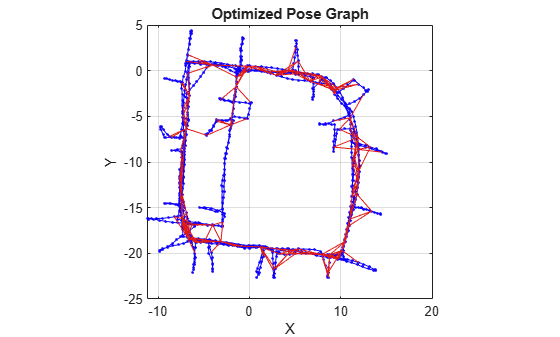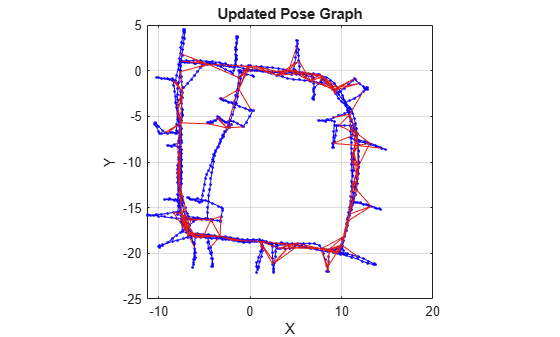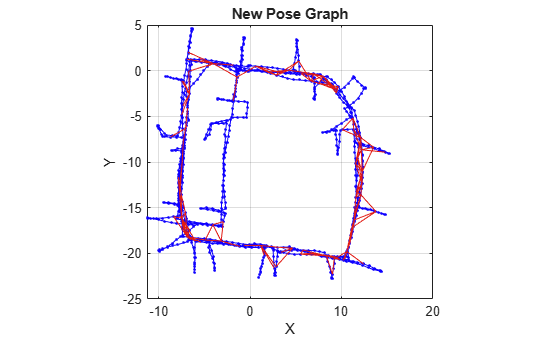# edgeConstraints

Edge constraints in pose graph

Since R2019b

## Syntax

``measurements = edgeConstraints(poseGraph)``
``[measurements,infoMats] = edgeConstraints(poseGraph)``
``[measurements,infoMats] = edgeConstraints(poseGraph,edgeIDs)``

## Description

````measurements = edgeConstraints(poseGraph)` lists all edge constraints in the specified pose graph as a relative pose.```
````[measurements,infoMats] = edgeConstraints(poseGraph)` also returns the information matrices for each edge. The information matrix is the inverse of the covariance of the pose measurement.```

example

````[measurements,infoMats] = edgeConstraints(poseGraph,edgeIDs)` returns edge constraints for the specified edge IDs.```

## Examples

collapse all

This example shows how to identify and remove spurious loop closures from pose graph. To do this, you can modify the relative pose of a loop closure edge and try optimizing the pose graph with and without removing the auto spurious loop closure and compare the results.

Load the Intel Research Lab Dataset that contains a 2-D pose graph. Optimize the pose graph. Plot the pose graph with IDs off. Red lines indicate loop closures identified in the dataset.

```load intel-2d-posegraph.mat pg optimizedPG = optimizePoseGraph(pg); show(optimizedPG,IDs="off"); title("Optimized Pose Graph")```Modify the relative pose of the loop closure edge `1386` to some random values.

```loopclosureId = 1386; nodePair = edgeNodePairs(optimizedPG,loopclosureId); [relPose,infoMat] = edgeConstraints(optimizedPG,loopclosureId); relPose(2) = -5; relPose(3) = 1.5; addRelativePose(optimizedPG,relPose,infoMat,nodePair(1),nodePair(2));```

Optimize the pose graph without auto loop closure trimming. Plot the optimized pose graph to see the poor adjustment of the nodes with loop closures.

```[updatedPG,solutionInfo] = optimizePoseGraph(optimizedPG); show(updatedPG,IDs="off"); title("Updated Pose Graph")```Certain loop closures should be trimmed from the pose graph. Use the `trimLoopClosures` function to trim these bad loop closures. Set the truncation threshold and maximum iterations for the trimmer parameters.

`trimParams = struct("TruncationThreshold",0.5,"MaxIterations",100);`

Generate solver options.

`solverOptions = poseGraphSolverOptions("g2o-levenberg-marquardt");`

Use the `trimLoopClosures` function with the trimmer parameters and solver options. Plot the new pose graph to see the bad loop closures were removed.

```[newPG,trimInfo] = trimLoopClosures(updatedPG,trimParams,solverOptions); show(newPG,IDs="off"); title("New Pose Graph")```## Input Arguments

collapse all

Pose graph, specified as a `poseGraph` or `poseGraph3D` object.

Edge IDs, specified as a vector of positive integers.

## Output Arguments

collapse all

Measurements between nodes, returned as an n-by-3 matrix or n-by-7 matrix.

For `poseGraph` (2-D), each row is an `[x y theta]` vector, which defines the relative xy-position and orientation angle, `theta`, of a pose in the graph. For landmark positions, `theta` is returned as `NaN`.

For `poseGraph3D`, each row is an ```[x y z qw qx qy qz]``` vector, which defines the relative xyz-position and quaternion orientation, ```[qw qx qy qz]```, of a pose in the graph.

Note

Many other sources for 3-D pose graphs, including `.g2o` formats, specify the quaternion orientation in a different order, for example, `[qx qy qz qw]`. Check the source of your pose graph data before adding nodes to your `poseGraph3D` object.

Information matrices, specified in compact form as a n-by-6 or n-by-21 matrix, where n is the number of poses in the pose graph.

Each row is the upper triangle of the square information matrix. An information matrix represents the uncertainty of the measurement. The matrix is calculated as the inverse of the covariance. If the measurement is an `[x y theta]` vector, the covariance matrix is a 3-by-3 of pairwise covariance calculations. Typically, the uncertainty is determined by the sensor model.

For `poseGraph` (2-D), each information matrix is a six-element vector. The default is `[1 0 0 1 0 1]`. For landmark nodes, the last three elements are returned as `NaN`.

For `poseGraph3D`, each information matrix is a 21-element vector. The default is ```[1 0 0 0 0 0 1 0 0 0 0 1 0 0 0 1 0 0 1 0 1]```.

## Version History

Introduced in R2019b